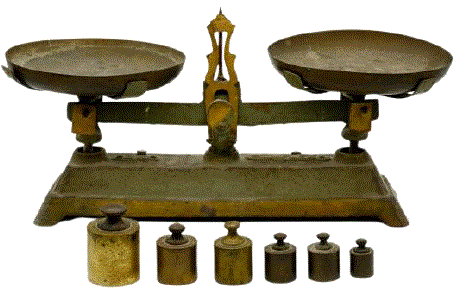Competitions

# Balance scaleOnce upon a time, scales and weights, which are almost never used today, were very widespread.

When the same masses are placed on both sides of the scale, they remain in equilibrium. Thus we know that the mass on both bowls is the same. You can measure different masses using weights, the masses of which are known in advance. Imagine that you have such scales, as well as n weights with integer masses. What is the smallest mass that cannot be measured with these scales and weights?

Suppose you have 4 weights with masses 1, 1, 4 and 15 kg. You can weigh 1 kg by placing one of the 1-kilogram weights on one scale pan. You can weigh 2 kg by placing two 1 -kg weights on one scale pan. 3 kg can be weighed by placing a 1-kilogram weight on one scale pan and a 4-kg weight on the other pan of the scale. The smallest mass that cannot be measured in this example is 7 kg.

#### Input

The first line contains one integer n (1n16) - the number of weights to be weighed. The second line contains n integers w1, w2, ..., wn (1wi108) - masses of weights.

#### Output

Print the smallest mass that cannot be measured with the balance and available weights.

Time limit 2 seconds
Memory limit 128 MiB
Input example #1
4
1 1 4 15

Output example #1
7

Input example #2
2
2 1

Output example #2
4

Input example #3
1
2

Output example #3
1

Input example #4
1
1

Output example #4
2

Source 2023 Azerbaijan, Semifinals, February 18, 8 - 9 classes